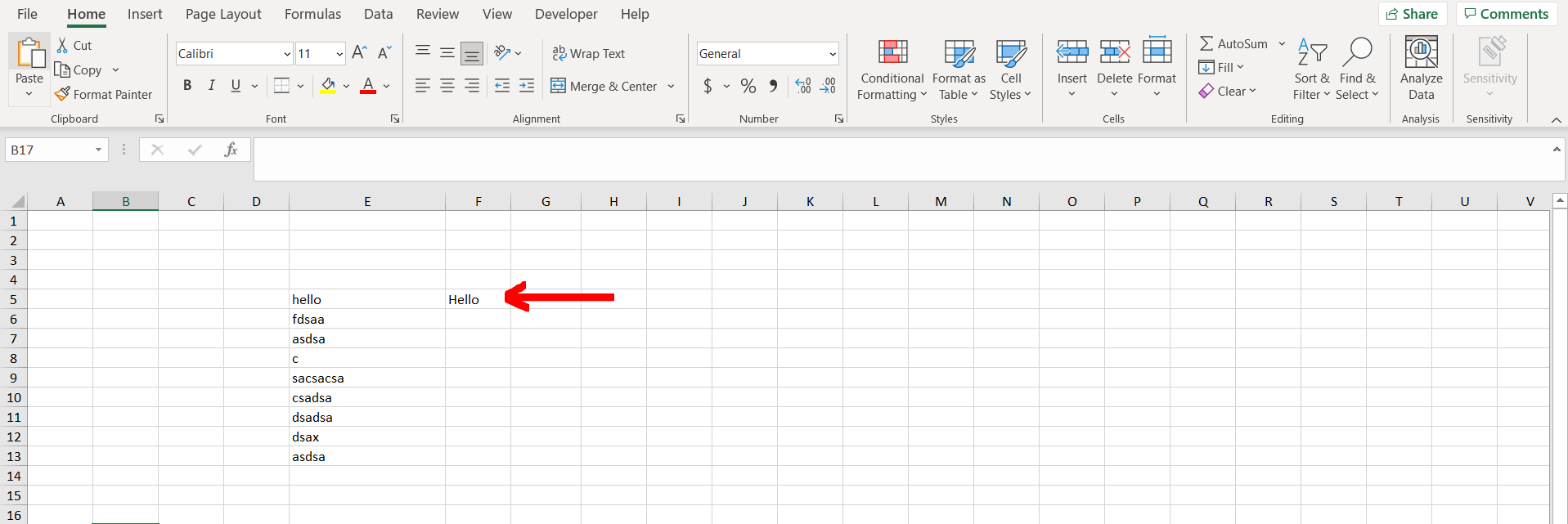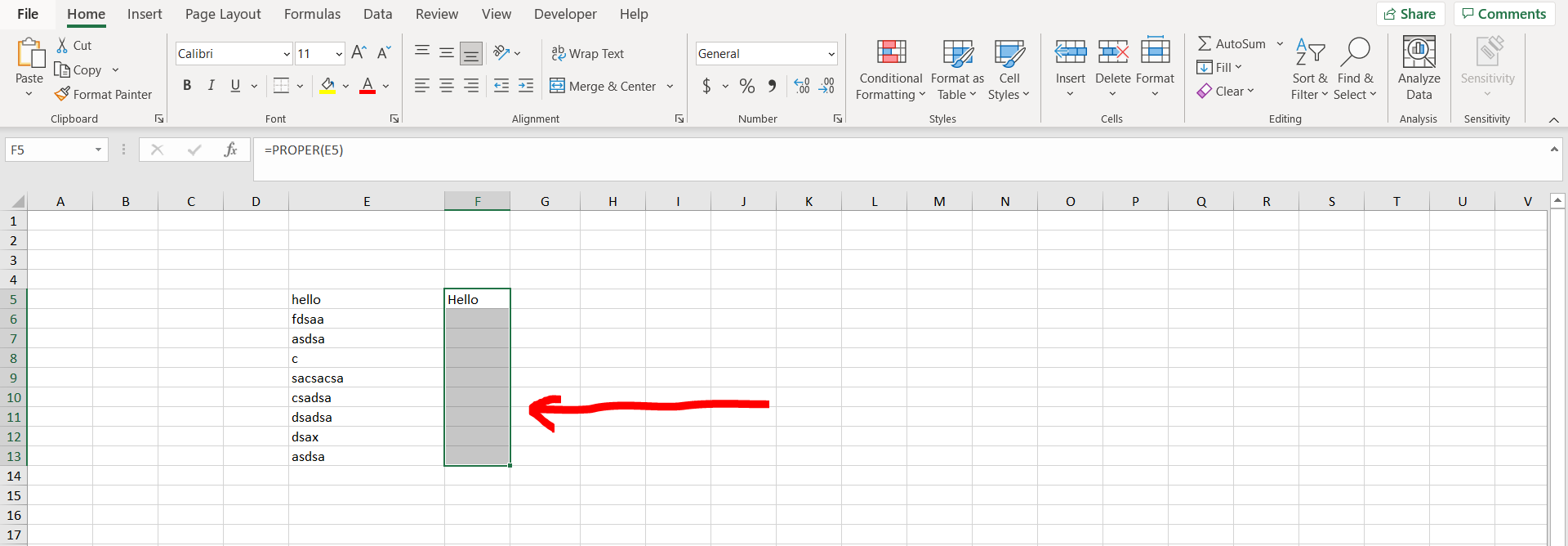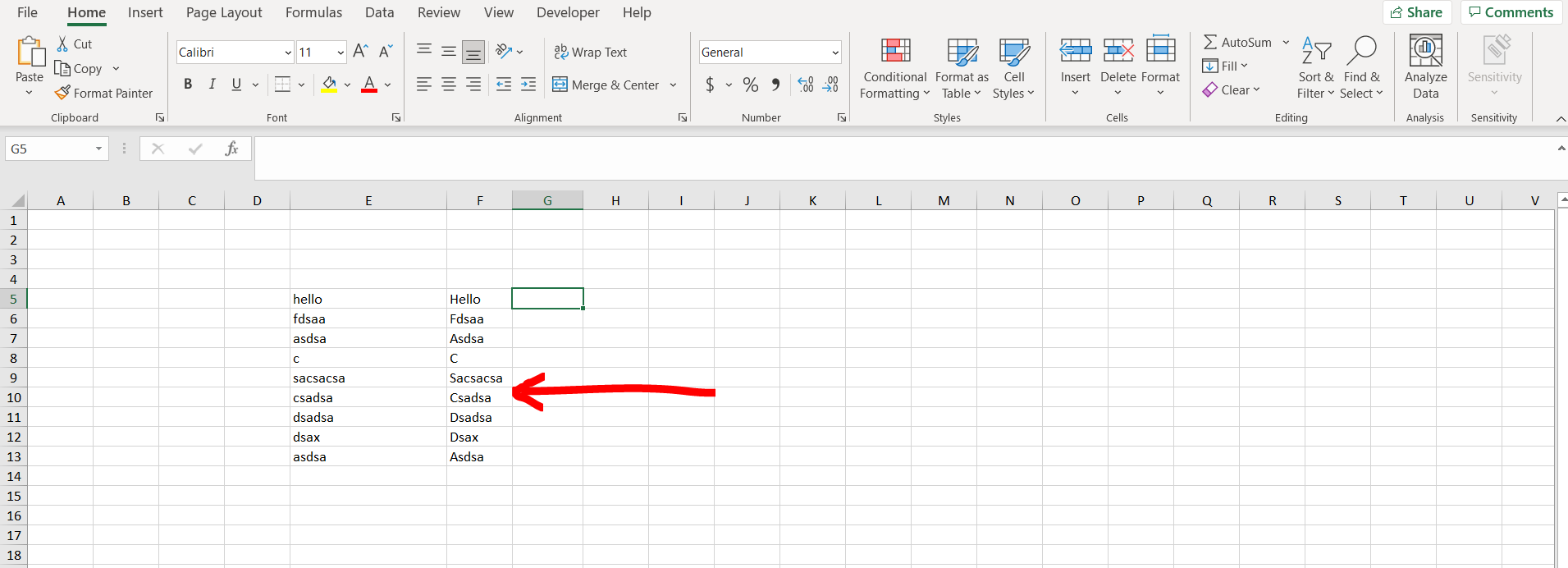# How to carry a formula down in ExcelExcel offers a very interesting way to carry a formula down. We can cater to this problem statement by copying the formula to the selection. We can perform the below mentioned way to carry a formula down in excel:

### Step 1 – Excel workbook with a column with some text– Open the desired Excel workbook which contains a column populated with some text, and adjacent to that write a formula corresponding to one cell.

### Step 2 – Entering the formula– Now copy the cell with the formula and select the number of cells till where you want to carry the formula, and then paste the copied cell.

### Step 3 – Formula carried down– We can see the formula has been carried down till our selection